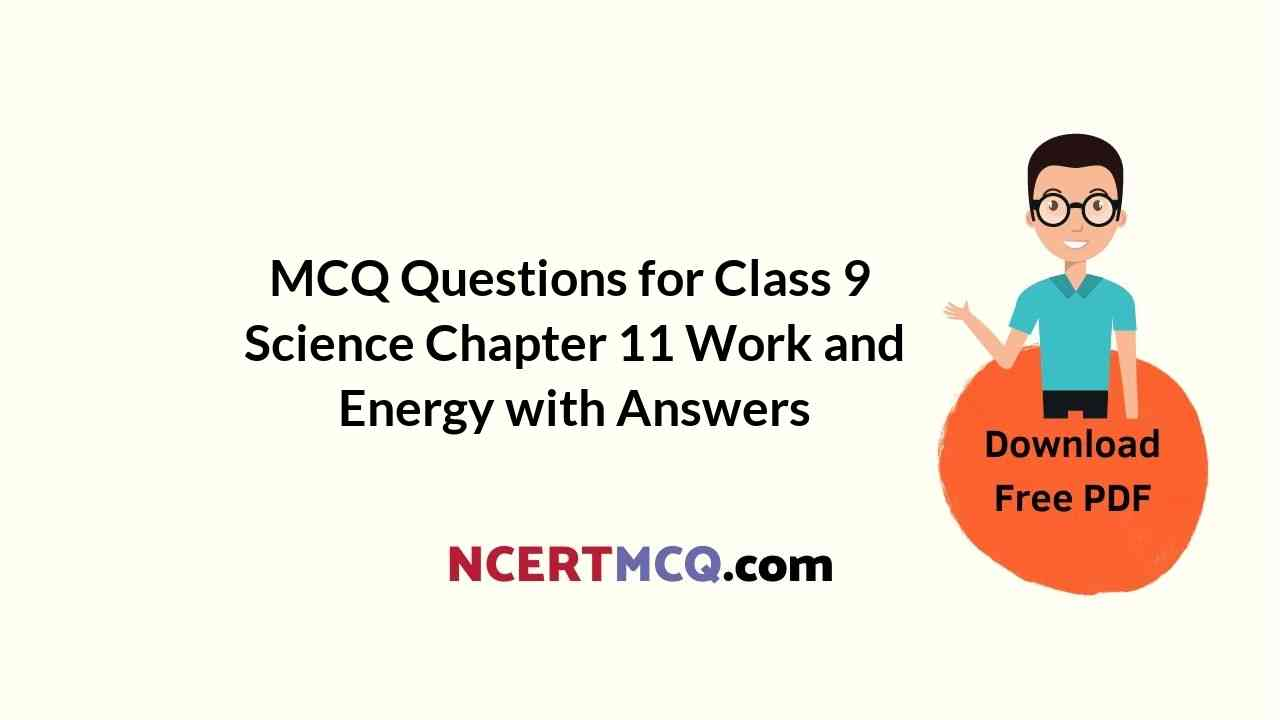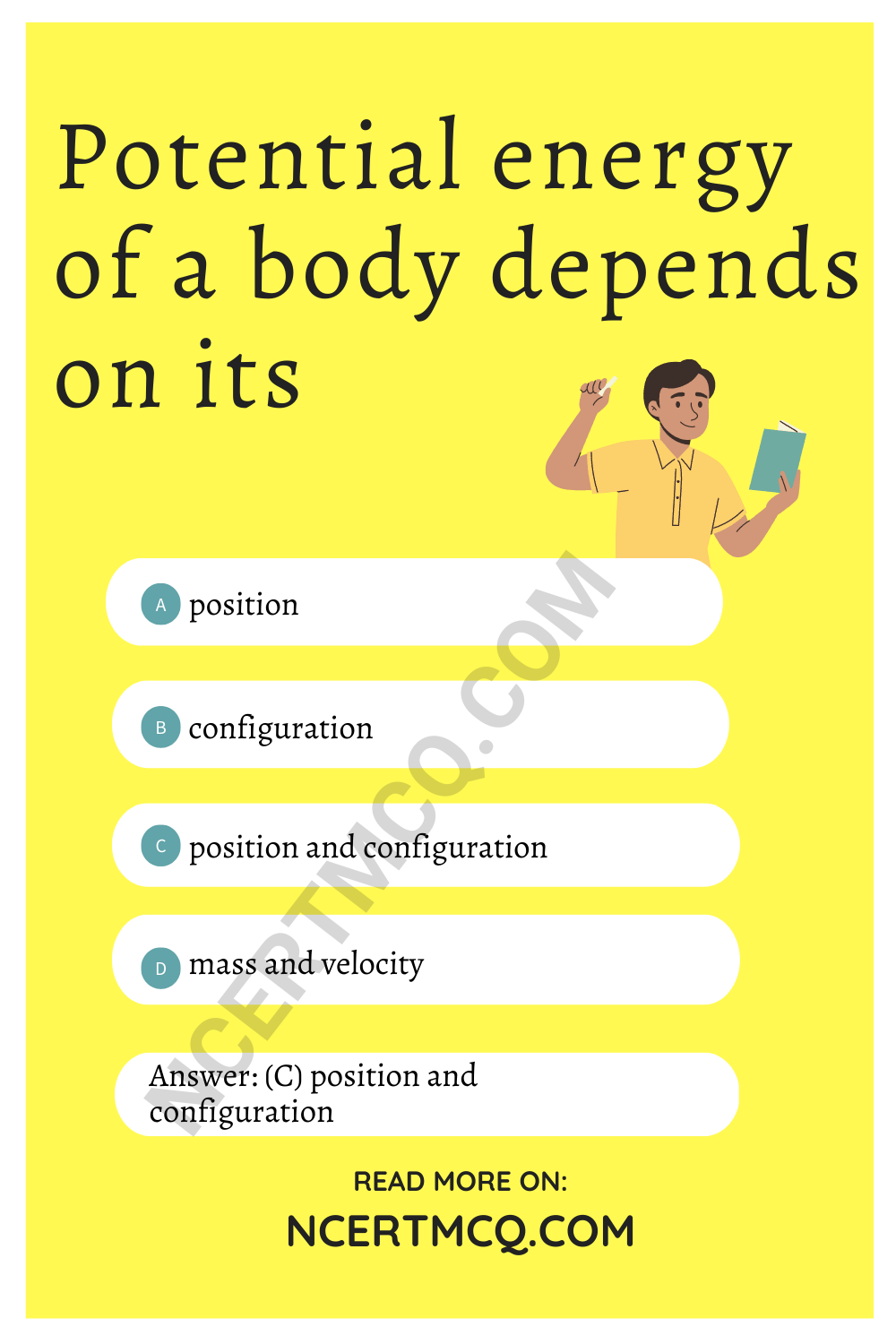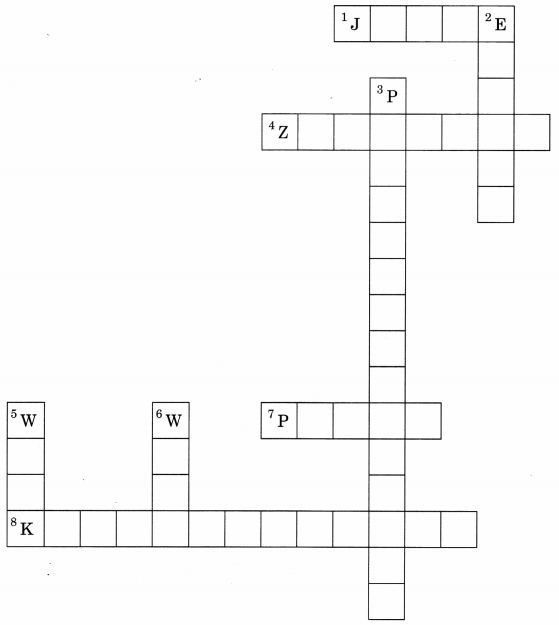Check the below Online Education NCERT MCQ Questions for Class 9 Science Chapter 11 Work and Energy with Answers Pdf free download. MCQ Questions for Class 9 Science with Answers were prepared based on the latest exam pattern. We have Provided Work and Energy Class 9 Science MCQs Questions with Answers to help students understand the concept very well. https://ncertmcq.com/mcq-questions-for-class-9-science-with-answers/

You can refer to NCERT Solutions for Class 9 Science Chapter 11 Work and Energy to revise the concepts in the syllabus effectively and improve your chances of securing high marks in your board exams.

## Work and Energy Class 9 MCQs Questions with Answers

Work And Energy Class 9 MCQ Question 1.
When a body falls freely towards the earth, then its total energy
(a) increases
(b) decreases
(c) remains constant
(d) first increases and then decreases

MCQ On Work And Energy Class 9 Question 2.
A car is accelerated on a levelled road and attains a velocity 4 times of its initial velocity. In this process the potential energy of the car
(a) does not change
(b) becomes twice to that of initial
(c) becomes 4 times that of initial
(d) becomes 16 times that of initial

Work And Energy Class 9 MCQ With Answers Question 3.
In case of negative work the angle between the force and displacement is (NCERT Exemplar)
(a) 0°
(b) 45°
(c) 90°
(d) 180°

Kinetic Energy Calculator is a handy tool that solves the kinetic energy problems easily & quickly.

Class 9 Work And Energy MCQ Question 4.
An iron sphere of mass 10 kg has the same diameter as an aluminium sphere of mass is 3.5 kg. Both spheres are dropped simultaneously from a tower. When they are lo m above the ground, they have the same.
(a) acceleration
(b) momenta
(c) potential energy
(d) kinetic energy

Class 9 Science Chapter 11 MCQ Question 5.
A girl is carrying a school bag of 3 kg mass on her back and moves 200 m on a levelled road. The work done against the gravitational force will be (g = 10 ms²)
(a) 6 × 10³ J
(b) 6 J
(c) 0.6 J
(d) zero

MCQ Of Work And Energy Class 9 Question 6.
Which one of the following is not the unit of energy?
(a) joule
(b) newton metre
(c) kilowatt
(d) kilowatt hour

Work And Energy MCQ Class 9 Question 7.
The work done on an object does not depend upon the
(a) displacement
(b) force applied
(c) angle between force and displacement
(d) initial velocity of the object

Answer: (d) initial velocity of the object

Work And Energy Class 9 MCQ Questions With Answers Question 8.
Water stored in a dam possesses
(a) no energy
(b) electrical energy
(c) kinetic energy
(d) potential energy

Class 9 Science Ch 11 MCQ Question 9.
A body is falling from a height h. After it has fallen a height $$\frac{h}{2}$$, it will possess
(a) only potential energy
(b) only kinetic energy
(c) half potential and half kinetic energy
(d) more kinetic and less potential energy

Answer: (c) half potential and half kinetic energyMCQ Work And Energy Class 9 Question 10.
The number of joules contained in 1 kWh is
(a) 36 × 105 J
(b) 3.6 × 107 J
(c) 36 × 108 J
(d) 3.7 × 107 J

Answer: (a) 36 × 105 J

Work Energy And Power Class 9 MCQ Question 11.
Two army persons A and B each of weight of 500 N climb up a rope through a height of 10 m. A takes 20 s while B takes 40 s to achieve this task. What is ratio of the powers of person A and B?
(a) 1 : 2
(b) 1 : 4
(c) 2 : 1
(d) 14 : 1

MCQ Questions On Work And Energy Class 9 Question 12.
Which of the following graphs best represents graphical relation between momentum P and kinetic energy K for a body in motion?Work And Energy Class 9 MCQ Online Test Question 13.
If speed of a car becomes 2 times, its kinetic energy becomes
(a) 4 times
(b) 8 times
(c) 16 times
(d) 12 times

Ch 11 Science Class 9 MCQ Question 14.
Work done by friction
(a) increases kinetic energy of body
(b) decreases kinetic energy of body
(c) increases potential energy of body
(d) decreases potential energy of body.

Answer: (b) decreases kinetic energy of body

MCQ On Work And Energy Class 9 Pdf Question 15.
When a coil spring is compressed, the work is done on the spring. The elastic potential energy
(a) increases
(b) decreases
(c) disappears
(d) remains unchanged

Question 16.
One joule work is said to be done when
(a) a force of 1 N displaces a body by 1 cm
(b) a force of 1 N displaces a body by 1 m
(c) a force of 1 dyne displaces a body by 1 m
(d) a force of 1 dyne displaces a body by 1 cm.

Answer: (b) a force of 1 N displaces a body by 1 m

Question 17.
SI unit of power is
(a) watt
(b) joule
(c) newton
(d) metre

Question 18.
Mechanical energy of a body includes
(a) kinetic energy only
(b) potential energy only
(c) kinetic energy and potential energy
(d) none of these

Answer: (c) kinetic energy and potential energy

Question 19.
Commercial unit of energy is
(a) joule
(b) kWh
(c) watt
(d) newton

Question 20.
Potential energy of a body depends on its
(a) position
(b) configuration
(c) position and configuration
(d) mass and velocityFill in the blanks

1. Work done is product of ………….. and distance moved the direction of the force.

2. If the angle between force and displacement is …………. then work done is said to be zero.

3. Energy of a body is defined as the capacity or …………. to do work.

4. Mechanical energy includes ………….. and ……………

5. Energy can neither be ………….. nor ………….. it can only transformed from one form to another.

6. ………….. is defined as the rate of doing work.

7. Commercial unit of energy is ………………

Match the following columns

 Column A Column B (a) SI unit of power (i) 1/2 mv2 (b) Kinetic energy (ii) The change of one form of energy into another (c) Potential energy (iii) watt (d) SI unit of work (iv) If applied force on an object and displacement is in opposite direction. (e) Negative work (v) Energy possessed by a body due to its position or configuration. (f) Power (vi) Joule (g) Expression of kinetic energy (vii) Energy possessed by a body due to its motion. (h) Transformation of energy (viii) Rate of doing work

 Column A Column B (a) SI unit of power (iii) watt (b) Kinetic energy (vii) Energy possessed by a body due to its motion. (c) Potential energy (v) Energy possessed by a body due to its position or configuration. (d) SI unit of work (vi) Joule (e) Negative work (iv) If applied force on an object and displacement is in opposite direction. (f) Power (viii) Rate of doing work (g) Expression of kinetic energy (i) 1/2 mv2 (h) Transformation of energy (ii) The change of one form of energy into another

Crossword Puzzle:Across:
1. SI unit of work
4. Work done if force is perpendicular to displacement
7. Rate of doing work
8. Energy possessed by a body due to virtue of its motion
Down:
2. Ability to do work
3. Energy possessed by a body due to virtue of its position or configuration
5. Product of force and displacement
6. SI unit of power

Across:
1. Joule
4. Zerowork
7. Power
8. Kinetic energy

Down:
2. Energy
3. Potential energy
5. Work
6. Watt

We hope the given NCERT MCQ Questions for Class 9 Science Chapter 11 Work and Energy with Answers Pdf free download will help you. If you have any queries regarding Work and Energy CBSE Class 9 Science MCQs Multiple Choice Questions with Answers, drop a comment below and we will get back to you soon.